# KSEEB Solutions for Class 9 Maths Chapter 13 Surface Area and Volumes Ex 13.6

In this chapter, we provide KSEEB SSLC Class 9 Maths Chapter 13 Surface Area and Volumes Ex 13.6 for English medium students, Which will very helpful for every student in their exams. Students can download the latest KSEEB SSLC Class 9 Maths Chapter 13 Surface Area and Volumes Ex 13.6 pdf, free KSEEB SSLC Class 9 Maths Chapter 13 Surface Area and Volumes Ex 13.6 pdf download. Now you will get step by step solution to each question.

## Karnataka Board Class 9 Maths Chapter 13 Surface Area and Volumes Ex 13.6

(Assume π = 227 unless stated otherwise)

Question 1.
The circumference of the base of a cylindrical vessel is 132 cm and its height is 25 cm. How many litres of water can it hold ? (1000 cm= 1 l)
Solution:
The circumference of the base of cylindrical vessel, C = 132 cm.
height, h = 25 cm
C = 2πr = 132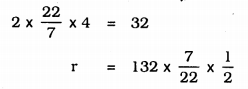r = 21 cm
∴ Volume of Cylinder, V = πr2h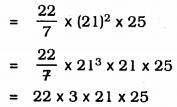= 34650 cm3
1000 cubic cm. = 1 litre
∴ 34650 cm3 = … ? …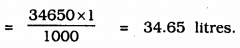Question 2.
The inner diameter of a cylindrical wooden pipe is 24 cm and its outer diameter is 28 cm. The length of the pipe is 35 cm. Find the mass of the pipe, if 1 cm3 of wood has a mass of 0.6 g.
Solution:
Let the inner diameter of a cylindrical vessel be d1 and outer diameter be d2.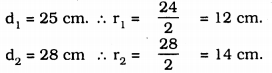height of pipe, h = 35 cm.
∴ volume of pipe, V = πr22h−πr21h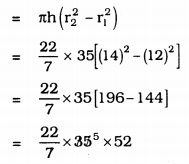= 110 × 52
∴ V = 5720 cm3
Mass of pipe of 1 ccm is 0.6 gm.
∴ Mass of 5720 cm3. … ? …
= 5720 × 0.6
= 3432 Kg.

Question 3.
A soft drink is available in two packs –
(i) a tin can with a rectangular base of length 5 cm and width 4 cm, having a height of 15 cm and
(ii) a plastic cylinder with circular base of diameter 7 cm and height 10 cm.
Which container has greater capacity and by how much ?
Solution:
Length of rectangular tin, l = 5 cm,
height, h = 15 cm.
∴ Volume of rectangular tin, V = l × b × h
V = 5 × 4 × 15
= 300 cm3
Diameter of plastic cylinder, d = 7 cm,
∴ r = 72
Volume of cylinder, v = πr2h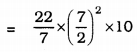= 11 × 7 × 5
= 385 cm3.
∴ Plastic cylinder’s volume is great.
= Volume of cylindrical tin – Volume of rectangular tin.
= 385 – 300
= 85 cm3.
∴ Volume of plastic tin is greater than rectangular tin by 85 cm3.

Question 4.
If the lateral surface of a cylinder is 94.2 cm2 and its height is 5 cm, then find
(ii) its volume. (Use π = 3.14)
Solution:
Curved surface area of cylinder = 94.2 cm2
height, h = 5 cm.
r = ?
V = ?
(i) Curved Surface area of cylinder, L.S.A.
A= 2πrh
94.2 = 2 × 3.14 × 5 × r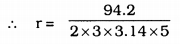= 3.01 cm.
= 3 cm.
(ii) Volume of cylinder, V = πr2h
= 3.14 × (3)2 × 5
= 3.14 × 9 × 5
= 141.3 cm3.

Question 5.
It costs Rs. 2200 to paint the inner curved surface of a cylindrical vessel 10 m deep. If the cost of painting is at the rate of Rs. 20 per m2, find
(i) inner curved surface area of the vessel.
(iii) capacity of the vessel.
Solution:
(i) Cost of painting for cylindrical vessel = Rs. 2200
Cost of painting is at the rate of Rs. 20 per m2.
∴ Curved Surface area of vessel = 220020
= 110 m2

(ii) Depth of vessel, h = 10 m
r = ?
Curved surface area of vessel, V = 2πrh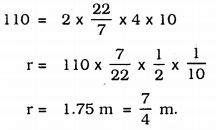(iii) Capacity of the Vessel = Volume of Vessel
V = πr2h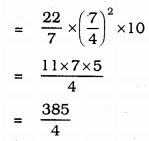V = 96.25 m3.

Question 6.
The capacity of a closed cylindrical vessel of height 1 m is 15.4 litres. How many square metres of metal sheet would be needed to make it ?
Solution:
Volume of a cylindrical vessel = 15.4 litres
1000 c.cm. = 1 litre.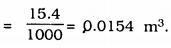height, h = 1 m,
Curved surface area =?
Volume of cylinder, V = πr2h
0. 0154 = 227 × r2 × 1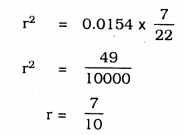r = 0.7 m
Now, curved surface area of cylinder vessel,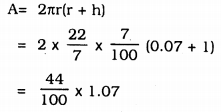= 0.44 × 1.07
A = 0.4708 m2

Question 7.
A lead pencil consists of a cylinder of wood with a solid cylinder of graphite filled in the interior. The diameter of the pencil is 7 mm and the diameter of the graphite is 1 mm. If the length of the pencil is 14 cm, find the volume of the wood and that of the graphite.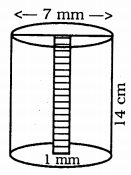Solution:
Diameter of pencil, d = 7 mm = 0.7 m.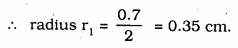length of pencil, h = 14 cm
diameter of pencil, d = 1 mm = 0.1 cm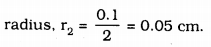Volume of wood in pencil, V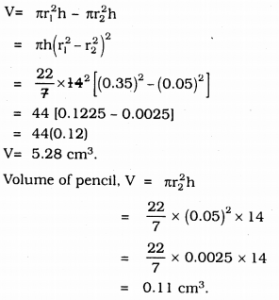Question 8.
A patient in a hospital is given soup daily in a cylindrical bowl of diameter 7 cm. If the bowl is filled with soup to a height of 4 cm, how much soup the hospital has to prepare daily to serve 250 patients?
Solution:
Diameter of pencil, d = 7 cm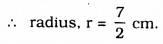height of soup, h = 4 cm
Volume of soup given for 1 patient is 154 cm3
Volume of soup given for 250 patients .. ? ….
= 154 × 250 = 38500 cm3
For 1000 cm3, 1 litre
For 38500 cm3 ….. ? …
= 385001000
= 38.5 litres soup.

All Chapter KSEEB Solutions For Class 9 Maths

—————————————————————————–

All Subject KSEEB Solutions For Class 9

*************************************************

I think you got complete solutions for this chapter. If You have any queries regarding this chapter, please comment on the below section our subject teacher will answer you. We tried our best to give complete solutions so you got good marks in your exam.

If these solutions have helped you, you can also share kseebsolutionsfor.com to your friends.

Best of Luck!!